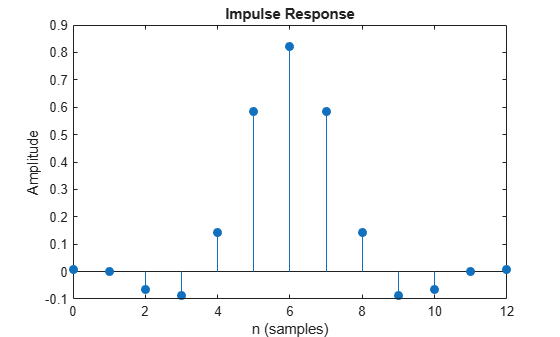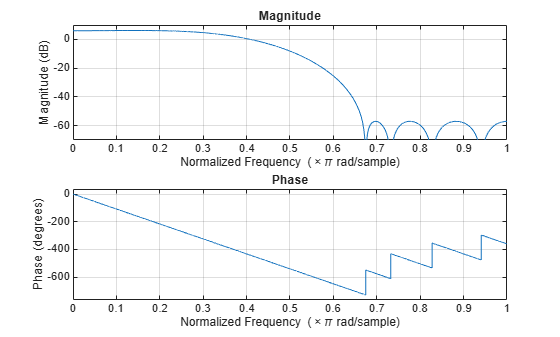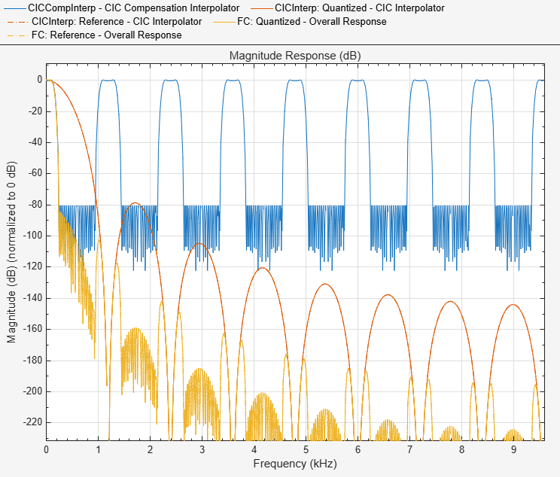# dsp.CICCompensationInterpolator

Compensate for CIC interpolation filter using FIR interpolator

## Description

You can compensate for the shortcomings of a CIC interpolator, namely its passband droop and wide transition region, by preceding it with a compensation interpolator. This System object™ lets you design and use such a filter.

To compensate for the shortcomings of a CIC filter using an FIR interpolator:

1. Create the `dsp.CICCompensationInterpolator` object and set its properties.

2. Call the object with arguments, as if it were a function.

## Creation

### Syntax

``ciccompint = dsp.CICCompensationInterpolator``
``ciccompint = dsp.CICCompensationInterpolator(interp)``
``ciccompint = dsp.CICCompensationInterpolator(cic)``
``ciccompint = dsp.CICCompensationInterpolator(cic,interp)``
``ciccompint = dsp.CICCompensationInterpolator(___,Name,Value)``

### Description

````ciccompint = dsp.CICCompensationInterpolator` returns a System object, `ciccompint`, that applies an FIR interpolator to each channel of an input signal. Using the properties of the object, the interpolation filter can be designed to compensate for a subsequent CIC filter.```
````ciccompint = dsp.CICCompensationInterpolator(interp)` returns a CIC compensation interpolator System object, `ciccompint`, with the `InterpolationFactor` property set to `interp`.```
````ciccompint = dsp.CICCompensationInterpolator(cic)` returns a CIC compensation interpolator System object, `ciccompint`, with the `CICRateChangeFactor`, `CICNumSections`, and `CICDifferentialDelay` properties specified in the `dsp.CICInterpolator` System object `cic`.```
````ciccompint = dsp.CICCompensationInterpolator(cic,interp)` returns a CIC compensation interpolator System object, `ciccompint`, with the `CICRateChangeFactor`, `CICNumSections`, and `CICDifferentialDelay` properties specified in the `dsp.CICInterpolator` System object `cic`, and the `InterpolationFactor` property set to `interp`.```

example

````ciccompint = dsp.CICCompensationInterpolator(___,Name,Value)` returns a CIC compensation interpolator object with each specified property set to the specified value. Enclose each property name in quotes. You can use this syntax with any previous input argument combinations.```

## Properties

expand all

Unless otherwise indicated, properties are nontunable, which means you cannot change their values after calling the object. Objects lock when you call them, and the `release` function unlocks them.

If a property is tunable, you can change its value at any time.

Specify the differential delay of the CIC filter being compensated as a positive integer scalar.

Data Types: `single` | `double` | `int8` | `int16` | `int32` | `int64` | `uint8` | `uint16` | `uint32` | `uint64`

Specify the number of sections of the CIC filter being compensated as a positive integer scalar.

Data Types: `single` | `double` | `int8` | `int16` | `int32` | `int64` | `uint8` | `uint16` | `uint32` | `uint64`

Specify the rate-change factor of the CIC filter being compensated as a positive integer scalar. The default is 2.

Data Types: `single` | `double` | `int8` | `int16` | `int32` | `int64` | `uint8` | `uint16` | `uint32` | `uint64`

Specify whether to design a filter of minimum order or a filter of specified order as a logical scalar. The default is `true`, which corresponds to a filter of minimum order.

Specify the order of the interpolation compensator filter as a positive integer scalar.

#### Dependencies

This property applies only when you set the `DesignForMinimumOrder` property to `false`.

Data Types: `single` | `double` | `int8` | `int16` | `int32` | `int64` | `uint8` | `uint16` | `uint32` | `uint64`

Specify the interpolation factor of the compensator System object as a positive integer scalar.

Data Types: `single` | `double` | `int8` | `int16` | `int32` | `int64` | `uint8` | `uint16` | `uint32` | `uint64`

Specify the passband edge frequency as a positive real scalar expressed in hertz. `PassbandFrequency` must be less than Fs/2, where Fs is the output sample rate.

Data Types: `single` | `double` | `int8` | `int16` | `int32` | `int64` | `uint8` | `uint16` | `uint32` | `uint64`

Specify the filter passband ripple as a positive real scalar expressed in decibels.

Data Types: `single` | `double` | `int8` | `int16` | `int32` | `int64` | `uint8` | `uint16` | `uint32` | `uint64`

Specify the input sample rate as a positive real scalar expressed in hertz.

Data Types: `single` | `double`

Specify the filter stopband attenuation as a positive real scalar expressed in decibels.

Data Types: `single` | `double` | `int8` | `int16` | `int32` | `int64` | `uint8` | `uint16` | `uint32` | `uint64`

Specify the stopband edge frequency as a positive real scalar expressed in hertz. `StopbandFrequency` must be less than Fs/2, where Fs is the output sample rate.

Data Types: `single` | `double` | `int8` | `int16` | `int32` | `int64` | `uint8` | `uint16` | `uint32` | `uint64`

### Fixed-Point Properties

Word and fraction lengths of coefficients, specified as a signed or unsigned `numerictype` object. The default, `numerictype(1,16)` corresponds to a signed numeric type object with 16-bit coefficients and a fraction length determined based on the coefficient values, to give the best possible precision.

This property is not tunable.

Word length of the output is same as the word length of the input. Fraction length of the output is computed such that the entire dynamic range of the output can be represented without overflow. For details on how the fraction length of the output is computed, see Fixed-Point Precision Rules for Avoiding Overflow in FIR Filters.

Rounding method for output fixed-point operations, specified as a character vector. For more information on the rounding modes, see Precision and Range.

## Usage

### Syntax

``y = ciccompint(x)``

### Description

example

````y = ciccompint(x)` outputs the upsampled and filtered values, `y`, of the input signal, `x`. ```

### Input Arguments

expand all

Data input, specified as a vector or a matrix. The System object treats a Ki × N input matrix as N independent channels, interpolating each channel over the first dimension.

This object does not support complex unsigned fixed-point data.

Data Types: `single` | `double` | `int8` | `int16` | `int32` | `int64` | `uint8` | `uint16` | `uint32` | `uint64` | `fi`
Complex Number Support: Yes

### Output Arguments

expand all

Upsampled and filtered signal, returned as a vector or matrix. For a Ki × N input matrix, the result is a Ko × N output matrix, where Ko = Ki × L and L is the interpolation factor.

Data Types: `single` | `double` | `int8` | `int16` | `int32` | `int64` | `uint8` | `uint16` | `uint32` | `uint64` | `fi`
Complex Number Support: Yes

## Object Functions

To use an object function, specify the System object as the first input argument. For example, to release system resources of a System object named `obj`, use this syntax:

`release(obj)`

expand all

 `freqz` Frequency response of discrete-time filter System object `fvtool` Visualize frequency response of DSP filters `info` Information about filter System object `cost` Estimate cost of implementing filter System object `coeffs` Returns the filter System object coefficients in a structure `polyphase` Polyphase decomposition of multirate filter `generatehdl` Generate HDL code for quantized DSP filter (requires Filter Design HDL Coder)
 `step` Run System object algorithm `release` Release resources and allow changes to System object property values and input characteristics `reset` Reset internal states of System object

## Examples

collapse all

Design an CIC compensation interpolator. Specify the interpolation factor to be 2, passband frequency to be 200 Hz, stopband frequency to be 500 Hz, and the input sample rate to be 600 Hz.

```fs = 600; fPass = 200; fStop = 500; CICCompInterp = dsp.CICCompensationInterpolator(... 'InterpolationFactor',2,... 'PassbandFrequency',fPass, ... 'StopbandFrequency',fStop,... 'SampleRate',fs);```

Plot the impulse response. The zeroth order coefficient is delayed 6 samples, which is equal to the group delay of the filter.

`fvtool(CICCompInterp,'Analysis','impulse')`Plot the magnitude and Phase response.

`fvtool(CICCompInterp,'Analysis','freq')`Note: If you are using R2016a or an earlier release, replace each call to the object with the equivalent step syntax. For example, `obj(x)` becomes `step(obj,x)`.

Design a compensation interpolator for an existing CIC interpolator having six sections and an interpolation factor of 16.

```CICInterp = dsp.CICInterpolator('InterpolationFactor',16, ... 'NumSections',6);```

Construct the compensation interpolator. Specify an interpolation factor of 2, an input sample rate of 600 Hz, a passband frequency of 100 Hz, and a stopband frequency of 250 Hz. Set the minimum attenuation of alias components in the stopband to be at least 80 dB.

```fs = 600; fPass = 100; fStop = 250; ast = 80; CICCompInterp = dsp.CICCompensationInterpolator(CICInterp, ... 'InterpolationFactor',2,'PassbandFrequency',fPass, ... 'StopbandFrequency',fStop,'StopbandAttenuation',ast, ... 'SampleRate',fs);```

Visualize the frequency response of the cascade. Normalize all magnitude responses to 0 dB.

```FC = dsp.FilterCascade(CICCompInterp, CICInterp); f = fvtool(CICCompInterp,CICInterp,FC, ... 'Fs', [fs*2 fs*16*2 fs*16*2]); f.NormalizeMagnitudeto1 = 'on'; legend(f,'CIC Compensation Interpolator','CIC Interpolator', ... 'Overall Response');```Apply the design to a 1000-sample random input signal.

```x = dsp.SignalSource(fi(rand(1000,1),1,16,15),'SamplesPerFrame',100); y = fi(zeros(32000,1),1,32,20); for ind = 1:10 x2 = CICCompInterp(x()); y(((ind-1)*3200)+1:ind*3200) = CICInterp(x2); end```

## Algorithms

The response of a CIC filter is given by:

`${H}_{cic}\left(\omega \right)={\left[\frac{\mathrm{sin}\left(\frac{RD\omega }{2}\right)}{\mathrm{sin}\left(\frac{\omega }{2}\right)}\right]}^{N}$`

R, D, and N are the rate change factor, the differential delay, and the number of sections of the CIC filter, respectively.

After decimation, the cic response has the form:okay

`${H}_{cic}\left(\omega \right)={\left[\frac{\mathrm{sin}\left(\frac{D\omega }{2}\right)}{\mathrm{sin}\left(\frac{\omega }{2R}\right)}\right]}^{N}$`

The normalized version of this last response is the one that the CIC compensator needs to compensate. Hence, the passband response of the CIC compensator should take the following form:

`${H}_{ciccomp}\left(\omega \right)={\left[RD\frac{\mathrm{sin}\left(\frac{\omega }{2R}\right)}{\mathrm{sin}\left(\frac{D\omega }{2}\right)}\right]}^{N}\text{for}\text{\hspace{0.17em}}\omega \le {\omega }_{p}<\pi$`

where ωp is the passband frequency of the CIC compensation filter.

Notice that when ω/2R ≪ π, the previous equation for Hciccomp(ω) can be simplified using the fact that sin(x) ≅ x:

This previous equation is the inverse sinc approximation to the true inverse passband response of the CIC filter.

## Version History

Introduced in R2014b